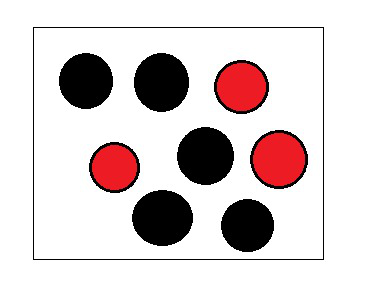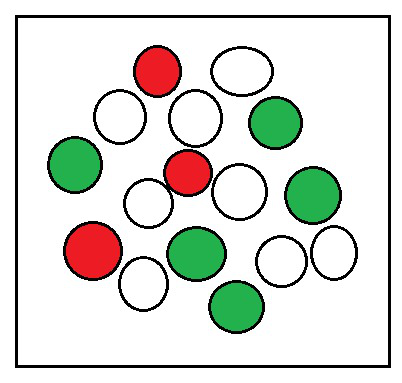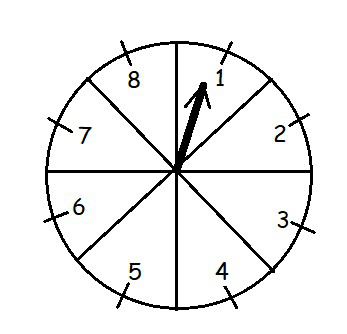GeeksforGeeks App
Open AppBrowser
Continue

# Class 10 NCERT Solutions- Chapter 15 Probability – Exercise 15.1 | Set 1

Theorem 1:

The theoretical probability (also called classical probability) of an event E, written as P(E), is defined as

P(E) =### Question 1. Complete the following statements:

(i) Probability of an event E + Probability of the event ‘not E’ =_________________ .

(ii) The probability of an event that cannot happen is __________. Such an event is called __________________ .

(iii) The probability of an event that is certain to happen is ______________. Such an event _______________ is called .

(iv) The sum of the probabilities of all the elementary events of an experiment is ______________________.

(v) The probability of an event is greater than or equal to _______________and less than or _________________ equal to .

Solution:

(i) 1

(ii) 0, Impossible Event

(iii) 1, Sure Event

(iv) 1

(v) 0, 1

### Question 2. Which of the following experiments have equally likely outcomes? Explain.

(i) A driver attempts to start a car. The car starts or does not start.

(ii) A player attempts to shoot a basketball. She/he shoots or misses the shot.

(iv) A baby is born. It is a boy or a girl.

Solution:

(i) This statement does not have equally likely outcomes as the car may or may not start depending upon various internal and external factors like fuel, path etc.

(ii) This statement does not have equally likely outcomes as the player may shoot or miss the shot depending upon various factors.

(iii) This statement has equally likely outcomes as it is known that the solution is either right or wrong, both have equal chance to happen.

(iv) This statement also has equally likely outcomes as it is known that the newly born baby can either be a boy or a girl, both have equal chance to happen.

### Question 3. Why is tossing a coin considered to be a fair way of deciding which team should get the ball at the beginning of a football game?

Solution:

Tossing of a coin is a fair way of deciding because they have equally likely outcomes where the number of possible outcomes are only 2 i.e. either head or tail. Tossing is considered to be completely unbiased and is unpredictable .

### Question 4. Which of the following cannot be the probability of an event?

(A) 2/3

(B) -1.5

(C) 15%

(D) 0.7

Solution:

As the probability of any event (E) always lies between 0 and 1

i.e. 0 ≤ P(E) ≤ 1

(i) 2/3 can be the probability of an event.

(ii) -1.5 cannot be the probability of an event.

(iii) 15% can be the probability of an event.

(iv) 0.7 can be the probability of an event.

### Question 5. If P(E) = 0.05, what is the probability of ‘not E’?

Solution:

As, P(E) + P(not E) = 1

P(Not E) = 1 – P(E) (Where the event ‘not E’ is complement of the event E)

Here, P(E) = 0.05

Then, P(Not E) = 1 – 0.05

P(Not E) = 0.95

### Question 6. A bag contains lemon-flavored candies only. Malini takes out one candy without looking into the bag. What is the probability that she takes out

(i) an orange flavoured candy?

(ii) a lemon flavoured candy?

Solution:

(i) As we know that the bag contains lemon-flavored candies only.

Hence, the no. of orange flavored candies = 0

The probability of taking out orange flavored candies =…………………… (from Theorem 1)

= 0 (Impossible Event)

(ii) As there are only lemon flavored candies,

P(Lemon flavored candies) = 1 (Sure Event)

### Question 7. It is given that in a group of 3 students, the probability of 2 students not having the same birthday is 0.992. What is the probability that the 2 students have the same birthday?

Solution:

As, P(E) + P(not E) = 1

P(E) = 1 – P(Not E) (Where the event ‘not E’ is complement of the event E)

Here, P(Not E) = Probability of 2 students not having the same birthday = 0.992

Here, P(E) = Probability that the 2 students have the same birthday

Then, P(E) = 1 – 0.992

P(E) = 0.08

Hence, the probability that the 2 students have the same birthday is 0.008

### Question 8. A bag contains 3 red balls and 5 black balls. A ball is drawn at random from the bag. What is the probability that the ball drawn is

(i) red?

(ii) not red?Solution:

Number of Red balls = 3

Number of Black balls = 5

Total number of balls = 5+3 = 8

Hence, total number of possible outcomes = 8

(i) P(E) = Probability that the ball drawn is Red.

P(E) =……………..(From Theorem 1)

(ii) P(Not E) = Probability that the ball drawn is not Red

P(Not E) = 1 – P(E) (Where the event ‘not E’ is complement of the event E)

P(Not red) = 1-### Question 9. A box contains 5 red marbles, 8 white marbles, and 4 green marbles. One marble is taken out of the box at random. What is the probability that the marble taken out will be

(i) red?

(ii) white?

(iii) not green?Solution:

Number of Red balls = 5

Number of White balls = 8

Number of Green balls = 4

Total number of balls = 5+8+4 = 17

Hence, total number of possible outcomes = 17

(i) P(E) = Probability that the ball drawn is Red.

P(E) =…………….. (From Theorem 1)

(ii) P(E) = Probability that the ball drawn is White.

P(E) =…………….. (From Theorem 1)

(iii) Let P(E) = Probability that the ball drawn is not Green.

P(E) =………. (From Theorem 1)

P(Not E) = 1 – P(E) (Where the event ‘not E’ is complement of the event E)

P(Not Green) = 1-### Question 10. A piggy bank contains hundred 50p coins, fifty ₹ 1 coins, twenty ₹ 2 coins and ten ₹ 5 coins. If it is equally likely that one of the coins will fall out when the bank is turned upside down, what is the probability that the coin

(i) will be a 50 p coin?

(ii) will not be a ₹ 5 coin?

Solution:

Number of 50p coins = 100

Number of ₹1 coins = 50

Number of ₹2 coins = 20

Number of ₹5 coins = 10

Total number of coins = 100+50+20+10 = 180

Hence, total number of possible outcomes = 180

(i) P(E) = Probability that the coin will be a 50 p coin.

P(E) =…………….. (From Theorem 1)

(ii) Let P(E) = Probability that the coin will be a ₹5 coin.

P(E) =.……………. (From Theorem 1)

Then, P(Not E) = Probability that the coin will not be a ₹5 coin.

P(Not E) = 1 – P(E) (Where the event ‘not E’ is complement of the event E)

P(Not ₹5 coin) = 1-### Question 11. Gopi buys a fish from a shop for his aquarium. The shopkeeper takes out one fish at random from a tank containing 5 male fish and 8 female fish. What is the probability that the fish taken out is a male fish?

Solution:

Number of male fish = 5

Number of female fish = 8

Total number of fish in the tank = 5+8 = 13

Hence, total number of possible outcomes = 13

P(E) = Probability that the fish will be male.

P(E)………………. (From Theorem 1)

### Question 12. A game of chance consists of spinning an arrow that comes to rest pointing at one of the numbers 1, 2, 3, 4, 5, 6, 7, 8 (see Fig. 15.5 ), and these are equally likely outcomes. What is the probability that it will point at

(i) 8?

(ii) an odd number?

(iii) a number greater than 2?

(iv) a number less than 9?Solution:

Here, total number of possible outcomes = 8

(i) P(E) = Probability that pointer will point at 8.

P(E)………………. (From Theorem 1)

(ii) P(E) = Probability that pointer will point at an odd number.

Odd number = {1,3,5,7}

P(E) =………………. (From Theorem 1)

(iii) P(E) = Probability that pointer will point at a number greater than 2.

Numbers greater than 2 = {3,4,5,6,7,8}

P(E) =………………. (From Theorem 1)

(iv) P(E) = Probability that pointer will point at a number less than 9.

Numbers less than 9 = {1,2,3,4,5,6,7,8}

P(E) == 1 ………………. (From Theorem 1)

My Personal Notes arrow_drop_up
Related Tutorials